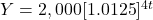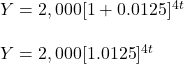Question

Write a function that represents the balance after t years.

$2000 deposit that earns 5% annual interest compounded quarterly y = Answers 1.trucchi Answer:Step-by-step explanation: Given: Amount deposit P =$2,000

Time t = t year (yearly) = 4t(quarterly)

Rate r = 5% annual = 5/4 = 1.25% (quarterly) = 0.0125

Find:

Amount after t year (Y)

Computation:

A = P[1+r]ⁿ

So,